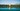# JavaScript 浮点数陷阱及解法

## 浮点数的存储

64 位比特又可分为三个部分：

• 符号位 S：第 1 位是正负数符号位（sign），0 代表正数，1 代表负数
• 指数位 E：中间的 11 位存储指数（exponent），用来表示次方数
• 尾数位 M：最后的 52 位是尾数（mantissa），超出的部分自动进一舍零### 为什么 `0.1 + 0.2 = 0.30000000000000004`？

``````// 0.1 和 0.2 都转化成二进制后再进行运算
0.0001100110011001100110011001100110011001100110011001101 +
0.001100110011001100110011001100110011001100110011001101 =
0.0100110011001100110011001100110011001100110011001100111

// 转成十进制正好是 0.30000000000000004``````

### 为什么 `x = 0.1` 能得到 `0.1`？

``````(0.10000000000000000555).toPrecision(16);
// 返回 0.1000000000000000，去掉末尾的零后正好为 0.1

// 但你看到的 `0.1` 实际上并不是 `0.1`。不信你可用更高的精度试试：
(0.1).toPrecision(21) = 0.100000000000000005551;``````

### 大数危机

``````Math.pow(2, 1023);
// 8.98846567431158e+307

Math.pow(2, 1024);
// Infinity``````

• `(2^53, 2^54)` 之间的数会两个选一个，只能精确表示偶数
• `(2^54, 2^55)` 之间的数会四个选一个，只能精确表示 4 个倍数
• ... 依次跳过更多 2 的倍数### `toPrecision` vs `toFixed`

• `toPrecision` 是处理精度，精度是从左至右第一个不为 0 的数开始数起。
• `toFixed` 是小数点后指定位数取整，从小数点开始数起。

## 解决方案

### 数据展示类

``parseFloat(1.4000000000000001.toPrecision(12)) === 1.4  // True``

``````function strip(num, precision = 12) {
return +parseFloat(num.toPrecision(precision));
}``````

### 数据运算类

``````/**
* 精确加法
*/
const num1Digits = (num1.toString().split('.') || '').length;
const num2Digits = (num2.toString().split('.') || '').length;
const baseNum = Math.pow(10, Math.max(num1Digits, num2Digits));
return (num1 * baseNum + num2 * baseNum) / baseNum;
}``````